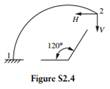Chat Now

# A cantilever circular arch rib with a uniform section is shown in Figure S24 A horizontal force H is applied to the free end of the rib so that end 2 can deflect only vertically when the vertical

### A cantilever circular arch rib with a uniform section is shown in Figure S24 A horizontal force H is applied to the free end of the rib so that end 2 can deflect only vertically when the vertical

A cantilever circular arch rib with a uniform section is shown in Figure S2.4 . A horizontal force H is applied to the free end of the rib so that end 2 can deflect only vertically when the vertical load V is applied at 2. Determine the ratio of H/V .rahul 09-Jul-2020Get solution